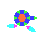1402164 Posts in 68045 Topics- by 61639 Members - Latest Member: pevogames

August 18, 2022, 11:43:57 PM

Need hosting? Check out Digital Ocean
TIGSource ForumsDeveloperTechnical(Moderator: ThemsAllTook)Generating fire and water in a Roguelike and Dijkstra MapsAuthor Topic: Generating fire and water in a Roguelike and Dijkstra Maps  (Read 987 times)
Gunroar:Cannon()« on: July 07, 2021, 08:28:02 AM »I was wondering how one would go about generatung the spreading of water and fire in a roguelike type view, turn by turn.
And also, how do Dijkstra maps work really. They turn a grid of paths into an array then work from there? Could I use it to generate fire? How do I set weights I can't find any java implementation, Thank youLogged

bayersglassey
Level 0« Reply #1 on: July 15, 2021, 10:32:56 PM »

http://www.roguebasin.com/index.php?title=Dijkstra_Maps_Visualized

As to how to generate the spread of water and fire turn by turn... cellular automata is the first thing to come to mind! The most famous ones like Conway's Game of Life use binary values, like "alive" or "dead", but you could use numbers too.

Basically, you want each cell on your grid to have some values, and then you want a rule for how those values change each turn, based on neighbouring cells' values.
For water, the easiest way to model it is probably an "amount" of water at each cell. But of course you could get tricky with it and model flowing water by storing a magnitude and direction of water movement at each cell, etc.

I'm not much of a Java person, but maybe something like this:
Code:
class Cell {
double waterAmount; // Number between 0 and some MAX_WATER_AMOUNT.

// addWaterAmount: reset to 0 at the start of each step, and added
// to waterAmount at the end of each step.

void takeWaterFrom(Cell other, double percentage) {
// Take a certain percentage of water from another cell.
// Remember, don't modify waterAmount directly!.. only ever modify
// That way you don't mess up any calculations which depend on current
// waterAmount values.

double takeWaterAmount = other.waterAmount * percentage;
}
}

class Map {
int width, height;
Cell[] cells;

Map(int width, int height) {
this.width = width;
this.height = height;
cells = new Cell[width * height];
}

Cell getCell(int x, int y) {
return cells[y * width + x];
}

void step() {
// Run a single "step" of the simulation.

// Update the addWaterAmount values of all cells.
// We do *NOT* visit the topmost and bottommost rows, nor the leftmost
// and rightmost columns, because those cells have fewer than 8 neighbours.
// There are various ways to deal with that, but this is probably the simplest.
// (That is, we start our loops of x and y from 1 instead of 0, and use
// "< width - 1" instead of "< width", etc.)
for(int y = 1; y < height - 1; y++) {
for(int x = 1; x < width - 1; x++) {
stepCell(x, y);
}
}

// Update waterAmount values, and reset addWaterAmount values.
int n_cells = width * height;
for(int i = 0; i < n_cells; i++) {
Cell cell = cells[i];
}
}

stepCell(int x, int y) {
// Modify the addWaterAmount value of the cell at (x, y) by looking at
// the waterAmount values of the neighbouring cells in a 3x3 grid around it.
// We give the neighbouring cells names, like this:
//
//   NW N NE
//
//   W     E
//
//   SW S SE

Cell cellNW = getCell(x - 1, y - 1);
Cell cellN  = getCell(x    , y - 1);
Cell cellNE = getCell(x + 1, y - 1);
Cell cellW  = getCell(x - 1, y    );
Cell cell   = getCell(x    , y    ); // <== current cell
Cell cellE  = getCell(x + 1, y    );
Cell cellSW = getCell(x - 1, y + 1);
Cell cellS  = getCell(x    , y + 1);
Cell cellSE = getCell(x + 1, y + 1);

// Now the following code is where you implement the rules
// which determine how your water behaves.
// (And if you added more values to Cell, like fire and whatnot,
// you could implement rules for those here as well.)

// Here's a really simple rule for how water could "spread":
// a certain percentage of it leaves each neighbouring cell and
// enters the current cell.
double percentage = .2;
cell.takeWaterFrom(cellNW, percentage);
cell.takeWaterFrom(cellN , percentage);
cell.takeWaterFrom(cellNE, percentage);
cell.takeWaterFrom(cellW , percentage);
cell.takeWaterFrom(cellE , percentage);
cell.takeWaterFrom(cellSW, percentage);
cell.takeWaterFrom(cellS , percentage);
cell.takeWaterFrom(cellSE, percentage);
}
}

I'm not sure if you already have a graphics library you want to use?..
But probably the best way to start playing with this stuff is to make a little demo where you can press a button and see one step at a time. And maybe add water by clicking with the mouse.

And by the way, in order to play with Djikstra maps, an easy way to start would be to add an "int distance" to class Cell...Logged
Gunroar:Cannon()« Reply #2 on: September 07, 2021, 02:35:20 PM »

Thanks!Logged